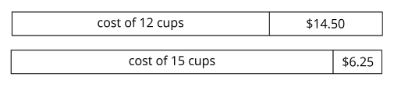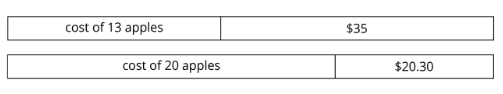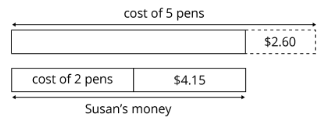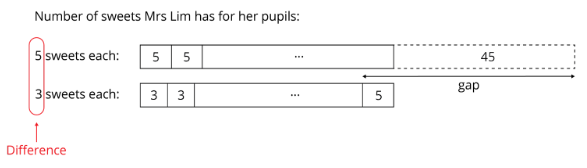Study P5 Mathematics Whole Number Strategy: Gaps & Differences - Geniebook# Whole Number Strategy: Gap & Difference

In this article, we are going to learn a strategy for Whole Numbers: Gap and Difference

The lesson objectives are:

1. Shortage/shortage
2. Excess/excess
3. Shortage/excess

## 1. Gap And Difference — Shortage/shortage

Do look at the models drawn and use it to help you solve the problem.

Question 1:

Sylvia wants to pack her game cards. If she packs $$8$$ cards into each packet, she will need another $$13$$ cards. If she packs $$7$$ cards into each packet, she will need another $$5$$ cards. How many cards does she have?

Solution:

In the above question, we have two scenarios.

Case 1:

She packs $$8$$ cards in each packet and has a shortage of $$13$$ cards.

Case 2:

She packs $$7$$ cards in each packet and has a shortage of $$5$$ cards.Difference $$= 8 - 7$$

$$= 1$$

Gap $$= 13 - 5$$

$$= 8$$

Number of packs $$=$$ Gap $$\div$$ Difference

$$​= 8 \div1 ​$$

$$= 8$$

Using Case 1:

Number of cards that she has $$= 8$$ packs $$8$$ cards $$- \;13$$

$$= 64 - 13$$

$$= 51$$ cards

Using Case 2:

Number of cards that she has  $$= 8$$ packs $$7$$ cards $$- \;5$$

$$= 54 - 5$$

$$= 51$$ cards

$$51$$ cards

Question 2:

A group of pupils share a box of pens among themselves. If each pupil gets $$6$$ pens, they will need another $$11$$ pens. If each pupil gets $$5$$ pens, they will need another $$4$$ pens.

1. How many pupils are there?
2. How many pens are there in the box?

Solution:

Case 1:

If each pupil gets $$6$$ pens, there is a shortage of $$11$$ pens.

Case 2:

if each pupil gets $$5$$ pens, there is a shortage of $$4$$ pens.A)

Difference $$= 6 - 5$$

$$= 1$$

Gap $$= 11 - 4$$

$$= 7$$

Number of pupils $$=$$ Gap $$\div$$ Difference

$$= 7 \div1$$

$$= 7$$

$$7$$ pupils

B)

Using Case 1:

Number of pens in the box $$= 7$$ pupils $$6$$ pens $$-\; 11$$

$$= 42 - 11$$

$$= 31$$ pens

Using Case 2:

Number of pens in the box $$= 7$$ pupils $$5$$ pens $$- \;4$$

$$= 35 - 4$$

$$= 31$$ pens

$$31$$ pens

Question 3:

Mrs Tan had some marbles to pack into a certain number of boxes. If she packed $$7$$ marbles into each box, the last box would have $$6$$ marbles. If she packed $$12$$ marbles into each box, the last box would have $$1$$ marble. How many boxes did she have?

Solution:

Case 1:

If she packed $$7$$ marbles in each box, there is a shortage of ( $$7-6=1$$ ) marble.

Case 2:

If she packed $$12$$ marbles in each box, there is a shortage of ( $$12-1=11$$ ) marbles.Difference $$= 12 - 7$$

$$= 5$$

Gap $$= 11 - 1$$

$$= 10$$

Number of boxes $$=$$ Gap $$\div$$ Difference

$$= 10-5$$

$$= 2$$

$$2$$ boxes

Question 4:

Keagan has $$7$$ boxes of chocolates to distribute to his friends. If he gives $$9$$ chocolates to each friend, he would be short of $$30$$ chocolates. If he gives $$8$$ chocolates to each friend, one of his friends would not get any chocolates. How many chocolates are there in each box?

Solution:

Total boxes of chocolates = $$7$$ boxes

Case 1:

If each friend gets $$9$$ chocolates, there is a shortage of $$30$$ chocolates.

Case 2:

If each friend gets $$8$$ chocolates, there is a shortage of $$8$$ chocolates since one friend did not get any chocolates.Difference $$= 9 - 8$$

$$= 1$$

Gap $$= 30 - 8$$

$$= 22$$

Number of friends $$=$$ Gap $$\div$$ Difference

$$= 22 \div 1$$

$$= 22$$

Using Case 1:

Number of chocolates $$= 22$$ friends $$× \;9$$ chocolates $$- \;30$$

$$= 198 - 30$$

$$= 168$$ chocolates

Using Case 2:

Number of chocolates $$= 22$$ friends $$× \;8$$ chocolates $$- \;8$$

$$= 176 - 8$$

$$= 168$$ chocolates

Number of chocolates in each box $$= 168 \div 7$$

$$= 24$$

$$24$$ chocolates

## 2. Gap And Difference — Excess/excess

Do look at the models drawn and use it to help you solve the problem.

Question 1:

Vicky has some money. If she buys $$12$$ cups, she will have $$14.50$$ left. If she buys $$15$$ cups, she will have $$6.25$$ left. How much does each cup cost?

Solution:

Case 1:

If she buys $$12$$ cups, she will have an excess of $$14.50$$.

Case 2:

If she buys $$15$$ cups, she will have an excess of $$6.25$$.Difference $$= 15 - 12$$

$$= 3$$

Gap $$= 14.50 - 6.25$$

$$= 8.25$$

Cost of 3 cups $$= 8.25$$

Cost of 1 cup $$= 8.25 \div 3$$

$$= 2.75$$

$$2.75$$

Question 2:

Mrs Mabel has some money. If she buys $$13$$ apples, she will have $$35$$ left. If she buys $$20$$ apples, she will have $$20.30$$ left. How much money does she have?

Solution:

Case 1:

If she buys $$13$$ apples, she has $$35$$ in excess.

Case 2:

If she buys $$20$$ apples, she has $$20.30$$ in excess.Difference $$= 20 - 13$$

$$= 7$$

Gap $$= 35 - 20.30$$

$$= 14.70$$

Cost of $$7$$ apples $$= 14.70$$

Cost of $$1$$ apple $$= 14.70 \div 7$$

$$= 2.10$$

Cost of $$20$$ apples $$= 20 \times 2.10$$

$$= 42$$

Amount of money Mrs Mabel has $$= 42 + 20.30$$

$$= 62.30$$

$$62.30$$

## 3. Gap And Difference — Shortage/excess

Do look at the models drawn and use it to help you solve the problem.

Question 1:

Susan wanted to buy $$5$$ pens but she needed $$2.60$$ more. She decided to buy $$2$$ pens instead and had $$4.15$$ left. Find the cost of a pen.

Solution:

Case 1:

If she buys $$5$$ pens, there is a shortage of $$2.60$$.

Case 2:

If she buys $$2$$ pens, there is an excess of $$4.15$$.Difference $$= 5 - 2$$

$$= 3$$

Gap $$= 4.15 + 2.60$$

$$= 6.75$$

Cost of $$3$$ pens $$= 6.75$$

Cost of $$1$$ pen $$= 6.75 \div 3$$

$$= 2.25$$

$$2.25$$

Question 2:

Mrs Lim has some sweets for her pupils. If she gives each pupil $$3$$ sweets, she will have $$5$$ sweets left. If she gives each pupil $$5$$ sweets, she will be short of $$45$$ sweets. How many pupils are there in the class?

Solution:

Case 1:

If she gives $$3$$ sweets to each pupil, there is an excess of $$5$$ sweets.

Case 2:

If she gives $$5$$ sweets to each pupil, there is a shortage of $$45$$ sweets.Difference $$= 5 - 3$$

$$=2$$

Gap $$= 5 + 45$$

$$= 50$$

Number of pupils $$= 50 ÷ 2$$

$$= 25$$

$$25$$

## Conclusion

In this article, we learnt about the Whole Numbers Strategy: Gap and Differences and how to use them while solving problems. Do look at the models drawn and use it to help you solve the problem.

### Gap and difference:

• Shortage/shortage
• Excess/excess
• Shortage/excess

Continue Learning
Volume Of A Liquid Decimals - Operations & Conversions
Ratio: Introduction Average - Formula
Percentage, Fractions And Decimals Whole Numbers
Strategy - Equal Stage Angle Properties
Table Rates Whole Number Strategy: Gap & Difference
Fractions - Addition & Subtraction Ratio Strategy: Repeated IdentityPrimaryPrimary 1Primary 2Primary 3Primary 4Primary 5EnglishMaths
Volume Of A Liquid
Decimals - Operations & Conversions
Ratio: Introduction
Average - Formula
Percentage, Fractions And Decimals
Whole Numbers
Strategy - Equal Stage
Angle Properties
Table Rates
Whole Number Strategy: Gap & Difference
Ratio Strategy: Repeated IdentitySciencePrimary 6SecondarySecondary 1Secondary 2Secondary 3Secondary 4